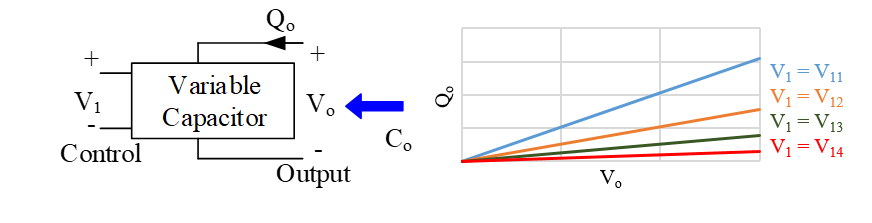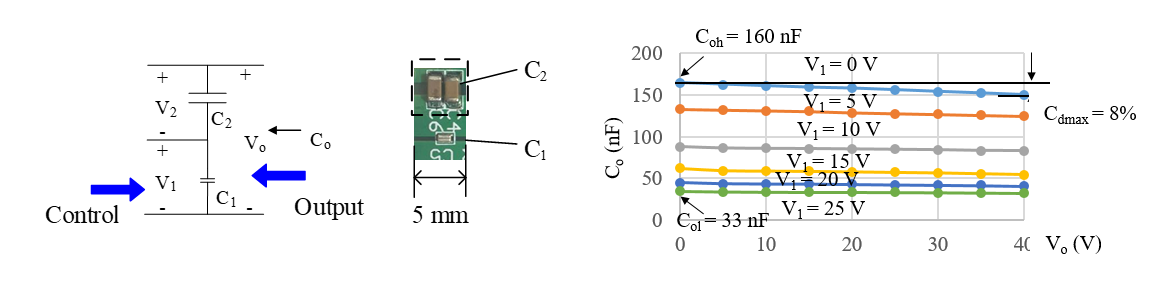LIBRARY

# Design and Characterization of a Linear Variable Capacitor

Year: 2018 | Author: Lujie Zhang | Paper: D5.2
Capacitors transfer power, store energy, and filter harmonics in power electronics applications. Steady-state performance (e.g., efficiency and voltage ripple) and dynamic performance (e.g., overshoot and settling time) of dc-dc converters are determined by capacitor properties such as capacitance, equivalent series resistance, voltage coefficient, and temperature coefficient. Capacitance usually drifts with the bias voltage because the permittivity of dielectric materials in a capacitor depends on the value of the applied electric field. Either a capacitor with stable permittivity (e.g., NP0) should be used, or the voltage across that capacitor should be well controlled to compensate for such undesired capacitance change, which both limits component selection and increases design complexity.

This paper presents the design of a linear variable capacitor (LVC), as shown in Fig. 1. LVC is defined when the output capacitance Co is only varied with the control voltage V1. The ideal Q-V curves of a LVC are shown in Fig. 1 (b). Straight lines demonstrate the linearity in the output, and each slope stands for different capacitances determined by V1.

The structure and the prototype of the designed LVC is shown in Fig. 2 (a) and Fig. 2 (b). C1 is a class II ceramic capacitor for controlling while C2 includes two ceramic capacitors for decoupling the effect of output voltage on output capacitor.

The performance of the designed LVC is shown in Fig. 2 (c). The value of Co is ranging from 33 nF to 160 nF so that the highest controllability is 382 percent. With a fixed V1, the highest decrease of Co is 8 percent, and the controllability drop is 16 percent within the whole Vo range. Future work will be focused on driver design and control strategy.Fig. 1. (a) Schematic of a variable capacitor and (b) Q-V curves of an ideal LVC.Fig. 2. (a) The structure and (b) the prototype and (c) the measured Co vs. Vo parametric with V1 under 500 kHz of LVC.